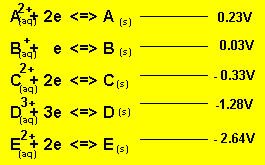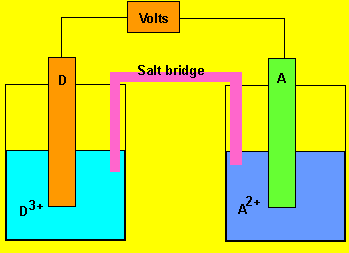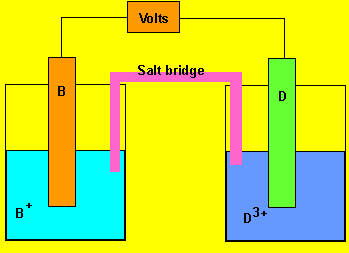Advanced exercisesSolution Using the reactivity series above, answer the following questions for each cell. a) What is the potential difference generated by the cell? b) In which direction do the electrons flow? c) What happens to the mass of each electrode ? d) Which of the two electrodes is the anode and which is the cathode? e) What charged particles flow into each half cell? f) Give the overall redox reactionSolutionSolution Continue